Courses

# Summary - Rigid Body: Force Systems Mechanical Engineering Notes | EduRev

## Mechanical Engineering : Summary - Rigid Body: Force Systems Mechanical Engineering Notes | EduRev

The document Summary - Rigid Body: Force Systems Mechanical Engineering Notes | EduRev is a part of the Mechanical Engineering Course Engineering Mechanics - Notes, Videos, MCQs & PPTs.
All you need of Mechanical Engineering at this link: Mechanical Engineering

A. MECHANICS

Mechanics can be defined as the branch of physics concerned with the state of rest or motion of bodies that subjected to the action of forces.

 Archimedes (287–212 BC), Galileo (1564–1642), Sir Issac Newton (1642–1727) and Einstein (1878–1955) have contributed a lot to the development of mechanics. Contributions by Varignon, Euler, D. Alembert are also substantial. The mechanics developed by these researchers may be grouped as(i) Classical mechanics/Newtonian mechanics(ii) Relativistic mechanics(iii) Quantum mechanics/Wave mechanics.Sir Issac Newton, the principal architect of mechanics, consolidated the philosophy and experimental findings developed around the state of rest and state of motion of the bodies and put forth them in the form of three laws of motion as well as the law of gravitation. The mechanics based on these laws is called Classical mechanics or Newtonian Mechanics.Albert Einstein proved that Newtonian mechanics fails to explain the behaviour of high speed (speed of light) bodies. He put forth the theory of Relativistic Mechanics.Schrödinger (1887–1961) and Broglie (1892–1965) showed that Newtonian mechanics fails to explain the behaviour of particles when atomic distances are concerned. They put forth the theory of Quantum Mechanics.

Classifications of Classical Mechanics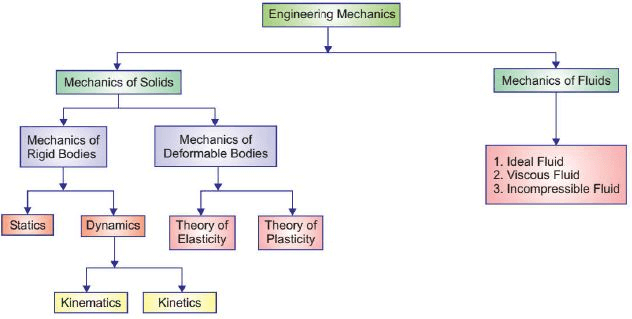B. RIGID BODY

A rigid body may be defined as a body in which the relative positions of any two particles do not change under the action of forces means the distance between two points/particles remain same before and after applying external forces.
OR
A body which does not deform under the influence of forces is known as a rigid body.
For a rigid body, relative positions of A’B’ and AB remains same before and after the application of forces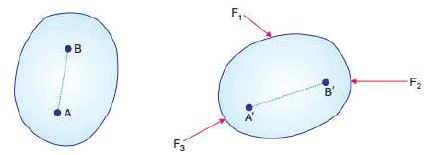Note :Physical bodies deform slightly under the action of loads. If the deformation is negligible compared to its size, the body is termed as rigid. In Rigid Body mechanics, we assume bodies to be rigid bodies.

Rigid Body Mechanics

Rigid Body Mechanics can be divided into two branches.

1. Statics: It is the branch of mechanics that deals with the study of forces acting on a body in equilibrium. Either the body at rest or in uniform motion is called statics

2. Dynamics: It is the branch of mechanics that deals with the study of forces on body in motion is called dynamics. It is further divided into two branches.

 Force Force may be defined as any action that tends to change the state of rest or motion of a body to which it is applied.The three quantities required to completely define force are called its specification or characteristics.1. Magnitude2. Point of application3. Direction of application/Line of actionForce is a vector quantity and its unit is Newton (N) in S.I. systems and dyne in C.G.S. system.Types Of Quantities 1. Scalar : Scalar quantities are those with which only a magnitude is associated. Examples of scalar quantities are time, volume, density, speed, energy, and mass2. Vector : Vector quantities, on the other hand, possess direction as well as magnitude, and must obey the parallelogram law of addition. Examples of vector quantities are displacement, velocity, acceleration, force, moment, and momentum.Effects of Force on a bodyWhenever a force acts on a body or particle, it may produce some external as well as internal effects or changes.A force may change the state or position of a body by inducing motion of the body. (External effect)A force may change the size or shape of an object when applied on it. It may deform the body thus inducing internal effects on the body.A force may induce rotational motion into a body when applied at a point other than its center of gravity.Line of action of forceThe direction of a force is the direction, along a straight-line through its point of application in which the force tends to move a body when it is applied. This line is called line of action of force.Graphical Representation of force Graphically a force may be represented by the segment of a straight line.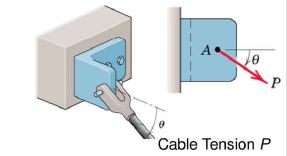C. LAWS OF MECHANICS

The following are the fundamental laws of mechanics:

1. Newton’s Laws of Motion

2. Newton’s Law of Gravitation

3. Law of transmissibility of forces

4. Parallelogram law of forces

Newton’s Laws of Motion

Law 1 :A particle remains at rest or continues to move with uniform velocity if there is no unbalanced force acting on it.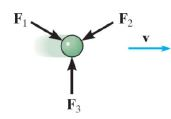Law 2 :The second law states that the rate of change of momentum of a body, is directly proportional to the force applied and this change in momentum takes place in the direction of the applied force.

Newton's second law of motion explains how an object will change velocity if acted by a force. For a body with constant mass, the second law can also be stated in terms of an object's acceleration. The acceleration of a particle is proportional to the vector sum of forces acting on it and occurs along a straight line in which the force acts.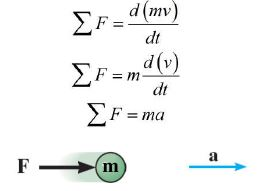Law 3 :To every action there is always an equal reaction: or the mutual interactions of two bodies are always equal but directed in opposite direction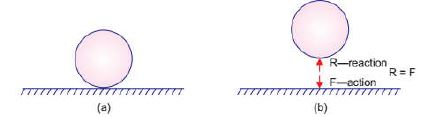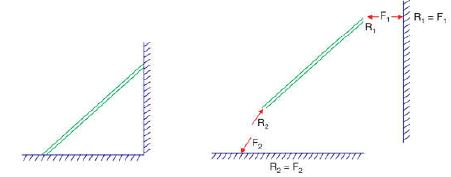Law of Gravitation

Two particles will be attracted towards each other along their connecting line with a force whose magnitude is directly proportional to the product of the masses and inversely proportional to the distance squared between the particles.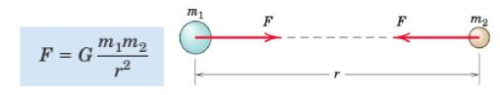Triangular law of forces “If the two forces acting on a body are represented in magnitude and direction as two sides of a triangle in order then the third side or the closing side of the triangle would be the resultant in opposite order.”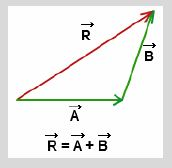Polygon Law of Forces If a number of concurrent forces acting on a rigid body are represented in magnitude and direction by the sides of a polygon, taken in order, then their resultant is represented in magnitude and direction by the closing side of polygon taken in reverse order.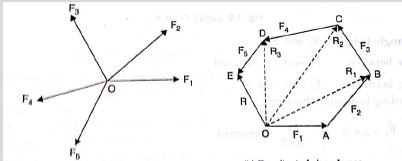Law of Transmissibility of Force

Principle of transmissibility states that a force may be applied at any point on a rigid body along its given line of action without altering the effects of the force on which it acts.
For example, the force F acts on a rigid body at point A. According to the principle of transmissibility of forces, this force has the same effect on the body as a force F applied at point B along its line of action.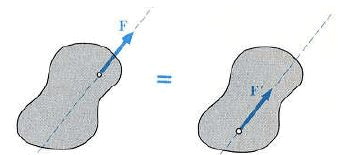Examples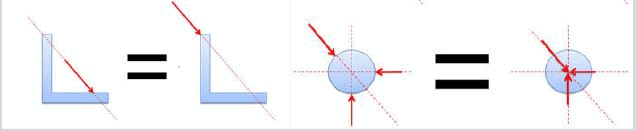Fig : Rigid Bodies (valid)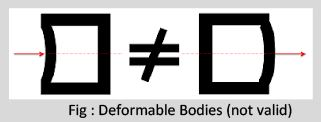Fig : Deformable Bodies (not valid) Note :In engineering mechanics we deal with only rigid bodies. If deformation of the body is to be considered in a problem, the law of transmissibility of forces will not hold good.

Parallelogram Law of Forces

If two forces acting at a point be represented in magnitude and direction by the two adjacent sides of a parallelogram, then their resultant is represented in magnitude and direction by lite diagonal of the parallelogram passing through that point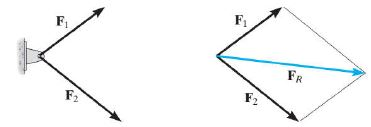Analytical Proof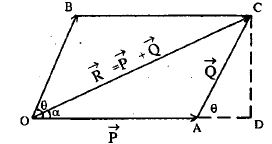Given OA = P force and OB = Q force. Construct parallelogram OBCA as shown and drop perpendicular CD on extension of OA.

Thus, AD= Q cos θ,CD=Q sin θ

OCD is a right angled triangle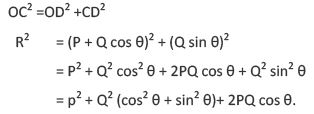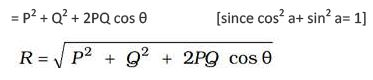Direction of R :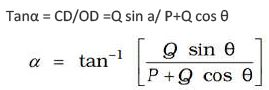Note : Parallelogram Law is valid for all vectors and hence forces also

D. FORCE SYSTEMS

When several forces of different magnitude and direction act upon a body or particle, it is called force system.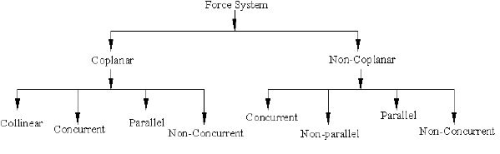TYPES OF FORCE SYSTEMS

1. Collinear Force System : When a system of forces act along the same line, they are called collinear forces.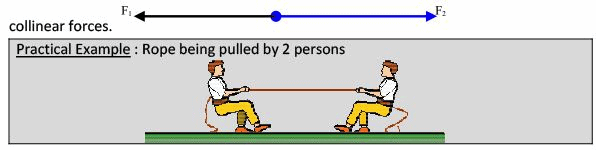2. Coplanar and Non Coplanar Force Systems

a. Coplanar Force Systems: When the system of forces are in a plane, it is called coplanar system of forces.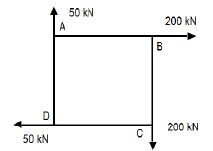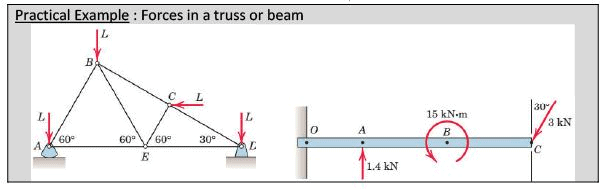b. Non-Coplanar Force System: When the system of forces are not in the same plane, it is called non-coplanar system of forces.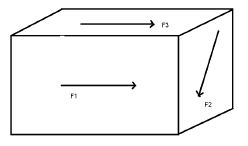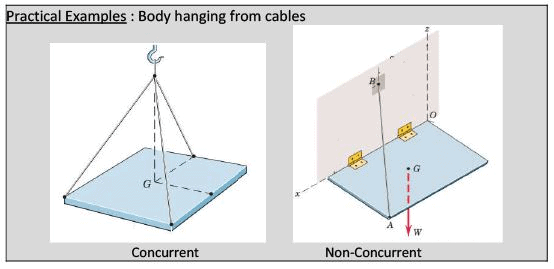3. Concurrent and Non Concurrent Force System: A concurrent force system contains forces whose lines-of action meet at same one point. They may either be

a. Coplanar Concurrent System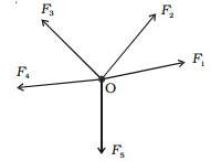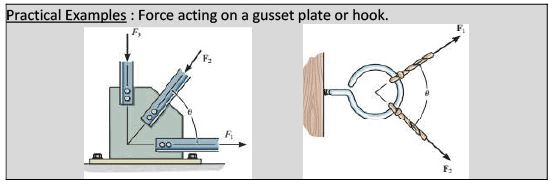b. Non-Coplanar Concurrent System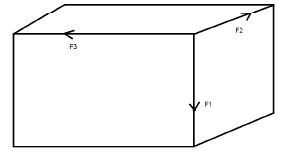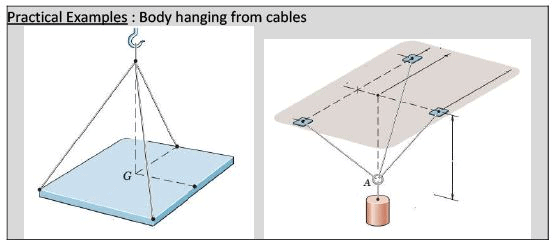4. Parallel Force Systems: Forces whose line of action are parallel to each other are called parallel force system. They are of 2 types

a. Like Parallel Forces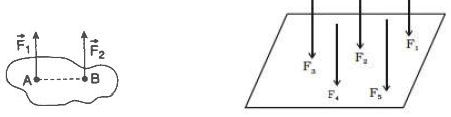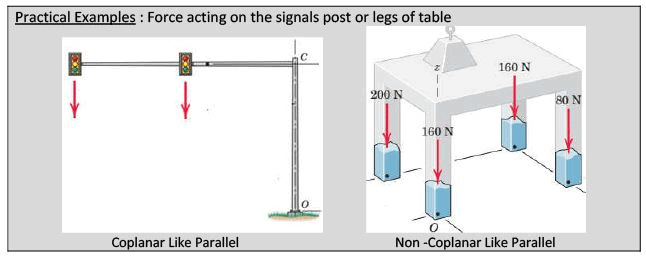b. Unlike Parallel Forces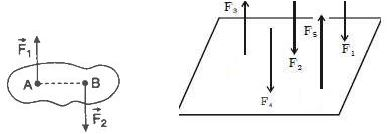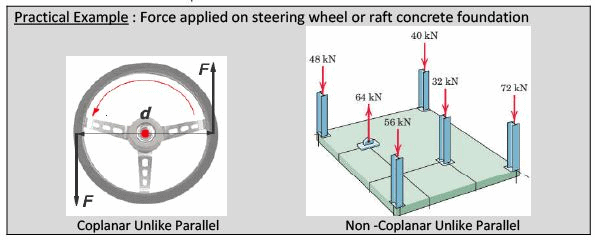Offer running on EduRev: Apply code STAYHOME200 to get INR 200 off on our premium plan EduRev Infinity!

,

,

,

,

,

,

,

,

,

,

,

,

,

,

,

,

,

,

,

,

,

;